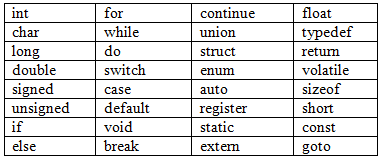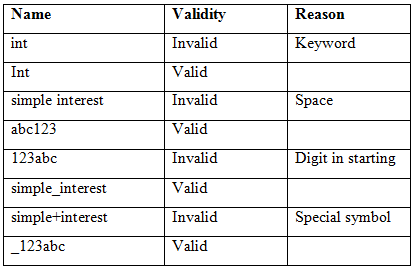## C Tokens

View

Tokens are the basic building blocks of C program. There are 5 types of tokens in C. They are-

1. Keywords
2. Identifiers
3. Literals (or Constants)
4. Operators
5. Separators

Keywords- There are some words that have special meaning to C. These are called reserve words, because they are reserve for C program. There are total 32 keywords in C. They are always written in small case alphabets. They are-Table 1: Keywords in C

Identifiers- These are names given to variables, functions, arrays, strings, structures etc.

Naming Rules for Identifiers - In real life, we can name our pet, our house or anything that we want to name and there is no specific rule for naming. Although, we take care that the name we choose do not hurt the sentiments of some other person.

But in C there are some rules that need to be followed for naming identifiers. They are-

1. It consist of alphabets, digits or underscore( _ ).
3. It can be up-to 8 characters long (in DOS) and 256 characters long (in Windows).
4. It should not be a Keyword.
5. It should not contain blank space.
6. Small case and capital case alphabets are distinct.
7. It should not contain special symbol (except underscore).

Example-Table 2: Example of identifiers

Literals- These are constant values that can be used in a C program. There are 4 types of literals in C. They are-

1. Integer Literals – For example- 10, 100, 23456 etc.
2. Float (Real) Literals – For example 10.4, 100.354 etc.
3. Character Literals – For example ‘a’, ‘+’, ‘1’ etc. It means any single character. They are always enclosed in single quotes.
4. String Literals - For example “college”, “a”, “what is your name?” etc. It means any group of characters or single character. They are always enclosed in double quotes.

Operators- These are symbols used to perform operation on operands. Operands may
be variables or constants values. For example-

A + B,    A+10 etc.

In above examples, A, B, 10 are operands and + is operator.

There are 3 categories of operators-

1. Unary operators - requires one operand. Example –

a++

-a

2. Binary operators - requires two operands. Example –

a+b,

a*b,

a-b

3. Ternary operators - requires three operands.Example – Conditional operator ( ? :)

operand1 ? operand2 : operand3

Various types of operators in C are-

1. Arithmetic operators
2. Assignment operator
3. Relational (or Comparison) operators
4. Logical operators
5. Increment and Decrement operators
6. Conditional operators
7. Bit-wise operators
8. Some special operators

We will discuss only Arithmetic operators and Assignment operator here. Rest of the operators will be discussed in other sections.

Arithmetic Operators- These operators are used to perform arithmetic operations. All arithmetic operators are binary except subtraction (-) which may be unary also. Various arithmetic operators in C are-

1. Addition ( + ) - For example- 10+20 = 30, 10.5+20 = 30.5

2. Subtraction ( - ) For example- 10-20 = -10, 10.5-20 = -9.5

3. Multiplication ( * ) For example- 10*20 = 200, 10.5*20 = 210.0

4. Division ( / )

Example-1. 10/20= 0 (because in C, integer/integer=integer)
Example-2. 10/20.0= 0.5 (because in C, integer/real=real)
Example-3. 10.0/20= 0.5 (because in C, real/integer=real)
Example-4. 10.0/20.0= 0.5 (because in C, real/real=real)

5. Modulus ( % )
This operator is used to find the remainder. Both of its operands must be integer.
Example-1. 10%3 = 1              Example-2. 10/3=3

Assignment Operator – There is only one assignment operator. Its symbol is ( = ). It is used to assign the value at its Right Hand Side (RHS) to the variable at its Left Hand Side (LHS). It is a binary operator.

Example- In Mathematics –

A = B + 20 (Correct)
B + 20 = A (Correct)

Example- In C language –

A = B + 20 (Correct)
B + 20 = A (Incorrect)

Compound assignment operators- These are mixture of arithmetic operators and assignment operator. They are binary operators. There are 5 compound assignment operators. They are-

1.      + =
2.      - =
3.      * =
4.      / =
5.      % =

Example-

A+ = 10 It means A = A + 10
A* = B It means A = A * B
And so on.

Separators- These are symbols used to separate a group of code from one another. The
most commonly used separator is a semicolon. As we have seen it is used to terminate the statement. Various separators in C are-

1. [ ] - Brackets
2. ( ) - Parentheses
3. { } - Braces
4. ;    - Semi Colon
5. .    - Period (Dot)
6. ,    - Comma